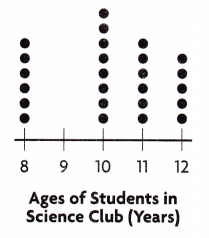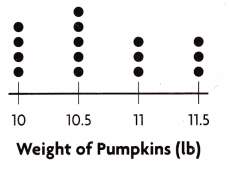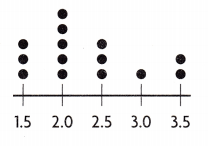# Texas Go Math Grade 5 Lesson 16.2 Answer Key Analyze Dot Plots

Refer to our Texas Go Math Grade 5 Answer Key Pdf to score good marks in the exams. Test yourself by practicing the problems from Texas Go Math Grade 5 Lesson 16.2 Answer Key Analyze Dot Plots.

## Texas Go Math Grade 5 Lesson 16.2 Answer Key Analyze Dot Plots

Unlock the Problem

You can identify the greatest and least numbers in a set of data on a dot plot.

Example 1
Members of a hiking club made a dot plot of the distances they hiked. What is the greatest distance they hiked? What is the least distance?The greatest number on the number line that has a dot above it is ___.
So, the greatest distance they hiked is ___ kilometers.
The least number on the number line that has a dot above it is ___.
So, the least distance they hiked is ___ kilometers.

The greatest number on the number line that has a dot above it is 9.5.
So, the greatest distance they hiked is 9.5 kilometers.
The least number on the number line that has a dot above it is 7.0.
So, the least distance they hiked is 7.0 kilometers.

The difference between the greatest and least numbers in a group is the range. What is the range of distances the hiking club hiked?

Subtract the least distance from the greatest distance.
___ km – ___ km = ___ km
The difference between the greatest and least distances
is ___ kilometers.
So, the range is ___ kilometers.
The least distance from the greatest distance is 9.5 – 7.0 = 2.5 km.
The difference between the greatest and least distances is 2.5 kilometers.
The range is 2.5 km.

Math talk

Mathematical Processes

Explain why 10.0 kilometer not used to find the range.

Example 2.
Solve a multi-step problem.
A breakfast chef uses three different amounts of milk when making pancakes, depending on the number of pancakes ordered. The dot plot shows customers’ orders for pancakes one morning. How much milk does the chef need to make all the pancakes?Step 1 Find the number of orders for pancakes that use each amount of milk.
___ dots above $$\frac{1}{4}$$: ___ orders that use $$\frac{1}{4}$$ cup of milk
___ dots above $$\frac{1}{2}$$: ___ orders that use $$\frac{1}{2}$$ cup of milk
___ dots above $$\frac{3}{4}$$: ___ orders that use $$\frac{3}{4}$$ cup of milk

2 dots above $$\frac{1}{4}$$: 2 orders that use $$\frac{1}{4}$$ cup of milk.
4 dots above $$\frac{1}{2}$$: 4 orders that use $$\frac{1}{2}$$ cup of milk.
2 dots above $$\frac{3}{4}$$: 2 orders that use $$\frac{3}{4}$$ cup of milk.

Explanation:
Here, there are 2 dots above $$\frac{1}{4}$$ and 2 orders that use $$\frac{1}{4}$$ cup of milk.
Here, there are 4 dots above $$\frac{1}{4}$$ and 4 orders that use $$\frac{1}{4}$$ cup of milk.
Here, there are 2 dots above $$\frac{3}{4}$$ and 2 orders that use $$\frac{3}{4}$$ cup of milk.

Step 2
Find the amount of milk needed for the orders that use each amount of milk.
$$\frac{1}{4}$$ × ___ = ___ cup
$$\frac{1}{2}$$ × ___ = ___ cup
$$\frac{3}{4}$$ × ___ = ___ cup

The amount of milk needed for the orders that use each amount of milk is $$\frac{1}{2}$$ cup, 2 cups and $$\frac{3}{2}$$ cup.

Explanation:
The amount of milk needed for the orders that use each amount of milk is
$$\frac{1}{4}$$ × 2 = $$\frac{1}{2}$$ cup.
$$\frac{1}{2}$$ × 4 = 2 cups.
$$\frac{3}{4}$$ × 2 = $$\frac{3}{2}$$ cup.

Step 3
Add to find the total amount of milk.
___ cup + ___ cups + ___ cups = __ cups
So, the chef needs ____ cups of milk to make all the pancakes.

The total amount of milk is 4 cups.
So, the chef needs 4 cups of milk to make all the pancakes.

Explanation:
The total amount of milk is $$\frac{1}{2}$$ + 2 + $$\frac{3}{2}$$
= $$\frac{1+4+3}{2}$$
= $$\frac{8}{2}$$
= 4 cups.
So, the chef needs 4 cups of milk to make all the pancakes.

Share and Show

Ana made a dot plot to show the ages of students in the science club. Use the dot plot for 1-3.Question 1.
How many more students are 10 years old than 12 years old?
number of dots above 10: ____
number of dots above 12: ____
___ – ___ = ____
The number of students who are 10 years old than 12 years old is 3 students.

Explanation:
The number of dots above 10 is 8 and
The number of dots above 12 is 5,
So the difference is 8 – 5 which is 3.

Question 2.
The same number of students are what two ages?
8 and 11.

Explanation:
The same number of students of two ages are 8 and 11.

Question 3.
What is the range of ages of students in the science club?
4.

Explanation:
The range of ages of students in the science club is 4.

Problem Solving

Use the dot plot for 4-5.

Question 4.
H.O.T. Communicate Describe a situation that could be represented by this dot plot. Give the dot plot a title.The title of the dot plot is The length of the movies.

Question 5.
Multi-Step Write and solve a problem that uses data from the dot plot.
How much time did I spend watching 2.25 hour movies than 3 hour movies?

Unlock the Problem

Question 6.
H.O.T. Multi-Step For 10 straight days, Samantha measured the amount of food that her cat Dewey ate, recording the results on a dot plot. What is the total amount of food that Dewey ate?a. What do you need to know?
We need to know the total amount of food Dewey ate.

b. What steps could you use to find the total amount of food that Dewey ate?First we will add the totals of each amount measured and then we will add those amount.

c. Fill in the blanks for the totals of each amount measured.The totals of each amount measured is
$$\frac{1}{4}$$ cup = $$\frac{1}{4}$$ × 2
= $$\frac{1}{2}$$ cup.
$$\frac{3}{8}$$ cup = $$\frac{3}{8}$$ × 1
= $$\frac{3}{8}$$ cup.
$$\frac{1}{2}$$ cup = $$\frac{1}{2}$$ × 3
= $$\frac{3}{2}$$ cups.
$$\frac{5}{8}$$ cup = $$\frac{5}{8}$$ × 3
= $$\frac{15}{8}$$ cups.
$$\frac{3}{4}$$ cup = $$\frac{3}{4}$$ × 1
= $$\frac{3}{4}$$ cup.

d. Find the total amount of cat food eaten over 10 days.
___ + ___ + ___ + ____ + __ = ___
So, Dewey ate ___ of cat food.
Dewey ate 5 cups of cat food.

Explanation:
The total amount of cat food eaten over 10 days is
$$\frac{1}{2}$$ + $$\frac{3}{8}$$ + $$\frac{3}{2}$$ + $$\frac{15}{8}$$ + $$\frac{3}{4}$$
= $$\frac{4+3+12+15+6}{8}$$
= $$\frac{40}{8}$$
= 5 cups.

Fill in the bubble completely to show your answer.

Question 7.
Communicate Allison is comparing prices of different cell phones. She made a dot plot to show the prices. How can she find the most common price?
(A) Find the dot all the way to the right.
(B) Find the dot all the way to the left.
(C) Find the shortest stack of dots.
(D) Find the tallest stack of dots.
D.

Explanation:
Find the tallest stack of dots.

Use the dot plot for 8-9.

Question 8.
How much do the greatest number of pumpkins weigh?
(A) 11 lb
(B) 10.5 lb
(C) 11.5 lb
(D) 10 lbB.

Explanation:
The greatest number of pumpkins weigh is 10.5 lb.

Question 9.
Multi-Step Jane bought all the pumpkins that weigh 10 pounds and 10.5 pounds. What is the total weight of the pumpkins she bought?
(A) 52.5 lb
(B) 92.5 lb
(C) 40 lb
(D) 81 lb
B.

Explanation:
The total weight of the pumpkins Jane bought is 10 × 4 + 10.5 × 5 which is 40 + 52.5 = 92.5 lb.

Texas Test Prep

Question 10.
The dot plot shows the heights of cornstalks at a farm. The same number of cornstalks are which two heights?(A) 5 ft and 5$$\frac{1}{2}$$ ft
(B) 4$$\frac{1}{2}$$ ft and 5 ft
(C) 4 ft and 4$$\frac{1}{2}$$ ft
(D) 4 ft and 5$$\frac{1}{2}$$ ft
B.

Explanation:
The same number of cornstalks with two heights are 4$$\frac{1}{2}$$ ft and 5 ft.

### Texas Go Math Grade 5 Lesson 16.2 Homework and Practice Answer Key

Students in Tony’s class made a dot plot of the number of letters in their first name. Use the dot plot for 1-3.

Question 1.
Write an equation to show how many students have 3, 4, or 5 letters in their name.The number equation is 4+6+5 = 15 letters.

Explanation:
The number of equation to show how many students have 3, 4, or 5 letters in their name is 4 + 6 + 5 which is 15 letters.

Question 2.
Do more students have first names with fewer than 5 letters or first names with more than 5 letters? Explain.

Question 3.
What is the range of the number of letters in the students’ first names? Show how you found your answer.

Problem Solving

Use the dot plot for 4-5.

Question 4.
Describe a real-world situation that could be represented by the dot plot. Write a title below the dot plot.Question 5.
Write and solve a problem that uses data from the dot plot.

Texas Teat Prep

Fill in the bubble completely to show your answer.

Question 6.
How long was the greatest number of phone calls?(A) 3$$\frac{1}{2}$$ min
(B) 3$$\frac{3}{4}$$ min
(C) 4min
(D) 4$$\frac{1}{2}$$ min
D.

Explanation:
The length of the greatest number of phone calls is 4$$\frac{1}{2}$$ min.

Question 7.
Nicki compares prices of movie tickets. She makes a dot plot to show the prices. How can Nicki find the price of the fewest number of movie tickets?
(A) Find the shortest stack of dots.
(B) Find the tallest stack of dots.
(C) Find the difference between the most expensive price and the least expensive price.
(D) Find the dot farthest to the left.

A.

Explanation:
Find the shortest stack of dots.Salads from the restaurant salad bar are sold by the ounce. A restaurant employee made a dot plot to show the weights of salads sold at lunchtime. Use the dot plot for 8-11.Question 8.
How many salads with a weight greater than 8.0 ounces were sold?
(A) 4
(B) 2
(C) 13
(C) 11
C.

Explanation:
The number of salads with a weight greater than 8.0 ounces were sold are 11 salads.

Question 9.
A total of five salads were sold at which two weights?
(A) 8.0 oz and 8.5 oz
(B) 8.0 oz and 9.0 oz
(C) 8.5 oz and 10 oz
(D) 9.5 oz and 10 oz
A.

Explanation:
The total of five salads were sold at which two weights is 8.0 oz and 8.5 oz.

Question 10.
Multi-Step The Ortega family bought all the salads that weigh 9.5 ounces and 10 ounces. What is the total weight of salads they bought?
(A) 30 0z
(B) 19.5 oz
(C) 39.5 oz
(D) 78 oz
Multi-Step The cost of salad per ounce is $0.50. How much did the restaurant collect for all the salads they sold that weighed 9.0 ounces? (A)$18
(B) $36 (C)$4.50
(D) $9.50 Answer: C. Explanation: Given that the cost of salad per ounce is$0.50, so the restaurant collect for all the salads they sold that weighed 9.0 is 9.0 × 0.50 which is \$4.50.